Normal view

# Applied logistic regression.

Material type:TextLanguage: English Edition: Third editionDescription: xvi, 500 pages : illustrations ; 24 cmISBN: 9780470582473 (hardback); 9781118514948 (oBook ISBN); 9781118514924 (ePDF ISBN); 9781118514931 (ePub ISBN); 9781118514900 (eMOBI ISBN)Additional physical formats: Online version:: Applied logistic regression.DDC classification: 519.536 HOA LOC classification: QA278.2 | .H67 2013Other classification: MAT029030 Online resources: Cover image | OCLC | E-book Fulltext
Contents:
Preface to the Third Edition xiii 1 Introduction to the Logistic Regression Model 1 1.1Introduction, 1 1.2 Fitting the Logistic Regression Model, 8 1.3 Testing for the Significance of the Coefficients, 10 1.4 Confidence Interval Estimation, 15 1.5 Other Estimation Methods, 20 1.6 Data Sets Used in Examples and Exercises, 22 1.6.1 The ICU Study, 22 1.6.2 The Low Birth Weight Study, 24 1.6.3 The Global Longitudinal Study of Osteoporosis in Women, 24 1.6.4 The Adolescent Placement Study, 26 1.6.5 The Burn Injury Study, 27 1.6.6 The Myopia Study, 29 1.6.7 The NHANES Study, 31 1.6.8 The Polypharmacy Study, 31 Exercises, 32 2 The Multiple Logistic Regression Model 35 2.1 Introduction, 35 2.2 The Multiple Logistic Regression Model, 35 2.3 Fitting the Multiple Logistic Regression Model, 37 2.4 Testing for the Significance of the Model, 39 2.5 Confidence Interval Estimation, 42 2.6 Other Estimation Methods, 45 Exercises, 46 3 Interpretation of the Fitted Logistic Regression Model 49 3.1 Introduction, 49 3.2 Dichotomous Independent Variable, 50 3.3 Polychotomous Independent Variable, 56 3.4 Continuous Independent Variable, 62 3.5 Multivariable Models, 64 3.6 Presentation and Interpretation of the Fitted Values, 77 3.7 A Comparison of Logistic Regression and Stratified Analysis for 2 × 2 Tables, 82 Exercises, 87 4 Model-Building Strategies and Methods for Logistic Regression 89 4.1 Introduction, 89 4.2 Purposeful Selection of Covariates, 89 4.2.1 Methods to Examine the Scale of a Continuous Covariate in the Logit, 94 4.2.2 Examples of Purposeful Selection, 107 4.3 Other Methods for Selecting Covariates, 124 4.3.1 Stepwise Selection of Covariates, 125 4.3.2 Best Subsets Logistic Regression, 133 4.3.3 Selecting Covariates and Checking their Scale Using Multivariable Fractional Polynomials, 139 4.4 Numerical Problems, 145 Exercises, 150 5 Assessing the Fit of the Model 153 5.1 Introduction, 153 5.2 Summary Measures of Goodness of Fit, 154 5.2.1 Pearson Chi-Square Statistic, Deviance, and Sum-of-Squares, 155 5.2.2 The Hosmer--Lemeshow Tests, 157 5.2.3 Classification Tables, 169 5.2.4 Area Under the Receiver Operating Characteristic Curve, 173 5.2.5 Other Summary Measures, 182 5.3 Logistic Regression Diagnostics, 186 5.4 Assessment of Fit via External Validation, 202 5.5 Interpretation and Presentation of the Results from a Fitted Logistic Regression Model, 212 Exercises, 223 6 Application of Logistic Regression with Different Sampling Models 227 6.1 Introduction, 227 6.2 Cohort Studies, 227 6.3 Case-Control Studies, 229 6.4 Fitting Logistic Regression Models to Data from Complex Sample Surveys, 233 Exercises, 242 7 Logistic Regression for Matched Case-Control Studies 243 7.1 Introduction, 243 7.2 Methods For Assessment of Fit in a 1--M Matched Study, 248 7.3 An Example Using the Logistic Regression Model in a 1--1 Matched Study, 251 7.4 An Example Using the Logistic Regression Model in a 1--M Matched Study, 260 Exercises, 267 8 Logistic Regression Models for Multinomial and Ordinal Outcomes 269 8.1 The Multinomial Logistic Regression Model, 269 8.1.1 Introduction to the Model and Estimation of Model Parameters, 269 8.1.2 Interpreting and Assessing the Significance of the Estimated Coefficients, 272 8.1.3 Model-Building Strategies for Multinomial Logistic Regression, 278 8.1.4 Assessment of Fit and Diagnostic Statistics for the Multinomial Logistic Regression Model, 283 8.2 Ordinal Logistic Regression Models, 289 8.2.1 Introduction to the Models, Methods for Fitting, and Interpretation of Model Parameters, 289 8.2.2 Model Building Strategies for Ordinal Logistic Regression Models, 305 Exercises, 310 9 Logistic Regression Models for the Analysis of Correlated Data 313 9.1 Introduction, 313 9.2 Logistic Regression Models for the Analysis of Correlated Data, 315 9.3 Estimation Methods for Correlated Data Logistic Regression Models, 318 9.4 Interpretation of Coefficients from Logistic Regression Models for the Analysis of Correlated Data, 323 9.4.1 Population Average Model, 324 9.4.2 Cluster-Specific Model, 326 9.4.3 Alternative Estimation Methods for the Cluster-Specific Model, 333 9.4.4 Comparison of Population Average and Cluster-Specific Model, 334 9.5 An Example of Logistic Regression Modeling with Correlated Data, 337 9.5.1 Choice of Model for Correlated Data Analysis, 338 9.5.2 Population Average Model, 339 9.5.3 Cluster-Specific Model, 344 9.5.4 Additional Points to Consider when Fitting Logistic Regression Models to Correlated Data, 351 9.6 Assessment of Model Fit, 354 9.6.1 Assessment of Population Average Model Fit, 354 9.6.2 Assessment of Cluster-Specific Model Fit, 365 9.6.3 Conclusions, 374 Exercises, 375 10 Special Topics 377 10.1 Introduction, 377 10.2 Application of Propensity Score Methods in Logistic Regression Modeling, 377 10.3 Exact Methods for Logistic Regression Models, 387 10.4 Missing Data, 395 10.5 Sample Size Issues when Fitting Logistic Regression Models, 401 10.6 Bayesian Methods for Logistic Regression, 408 10.6.1 The Bayesian Logistic Regression Model, 410 10.6.2 MCMC Simulation, 411 10.6.3 An Example of a Bayesian Analysis and Its Interpretation, 419 10.7 Other Link Functions for Binary Regression Models, 434 10.8 Mediation, 441 10.8.1 Distinguishing Mediators from Confounders, 441 10.8.2 Implications for the Interpretation of an Adjusted Logistic Regression Coefficient, 443 10.8.3 Why Adjust for a Mediator? 444 10.8.4 Using Logistic Regression to Assess Mediation: Assumptions, 445 10.9 More About Statistical Interaction, 448 10.9.1 Additive versus Multiplicative Scale--Risk Difference versus Odds Ratios, 448 10.9.2 Estimating and Testing Additive Interaction, 451 Exercises, 456 References 459 Index 479
Summary: "A new edition of the definitive guide to logistic regression modeling for health science and other applicationsThis thoroughly expanded Third Edition provides an easily accessible introduction to the logistic regression (LR) model and highlights the power of this model by examining the relationship between a dichotomous outcome and a set of covariables. Applied Logistic Regression, Third Edition emphasizes applications in the health sciences and handpicks topics that best suit the use of modern statistical software. The book provides readers with state-of-the-art techniques for building, interpreting, and assessing the performance of LR models. New and updated features include: A chapter on the analysis of correlated outcome data. A wealth of additional material for topics ranging from Bayesian methods to assessing model fit Rich data sets from real-world studies that demonstrate each method under discussion. Detailed examples and interpretation of the presented results as well as exercises throughout Applied Logistic Regression, Third Edition is a must-have guide for professionals and researchers who need to model nominal or ordinal scaled outcome variables in public health, medicine, and the social sciences as well as a wide range of other fields and disciplines"--Summary: "This Third Edition continues to focus on applications and interpretation of results from fitting regression models to categorical response variables"--
Tags from this library: No tags from this library for this title.Average rating: 0.0 (0 votes)
Item type Current location Collection Call number Copy number Status Date due Barcode Item holdsE-Book
E-book
Non-fiction 519.536 HOA 2013 (Browse shelf) Not For LoanText
Reserve Section
Non-fiction 519.536 HOA 2013 (Browse shelf) C-1 Not For Loan 25572Text
Reserve Section
Non-fiction 519.536 HOA 2013 (Browse shelf) C-2 Not For Loan 26667Text
Circulation Section
Non-fiction 519.536 HOA 2013 (Browse shelf) C-3 Available 26668
Total holds: 0
##### Browsing EWU Library shelves, Shelving location: E-book Close shelf browser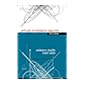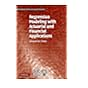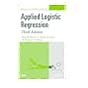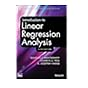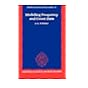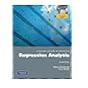519.536 DRA 2011 Applied regression analysis / 519.536 FRG 2010 Regression modeling with actuarial and financial applications / 519.536 HOA 2013 Applied logistic regression / 519.536 HOA 2013 Applied logistic regression. 519.536 INT 2012 Introduction to linear regression analysis / 519.536 LIM 1995 Modelling frequency and count data / 519.536 MES 2012 A second course in statistics :

Includes bibliographical references (pages 459-478) and index.

Preface to the Third Edition xiii 1 Introduction to the Logistic Regression Model 1 1.1Introduction, 1 1.2 Fitting the Logistic Regression Model, 8 1.3 Testing for the Significance of the Coefficients, 10 1.4 Confidence Interval Estimation, 15 1.5 Other Estimation Methods, 20 1.6 Data Sets Used in Examples and Exercises, 22 1.6.1 The ICU Study, 22 1.6.2 The Low Birth Weight Study, 24 1.6.3 The Global Longitudinal Study of Osteoporosis in Women, 24 1.6.4 The Adolescent Placement Study, 26 1.6.5 The Burn Injury Study, 27 1.6.6 The Myopia Study, 29 1.6.7 The NHANES Study, 31 1.6.8 The Polypharmacy Study, 31 Exercises, 32 2 The Multiple Logistic Regression Model 35 2.1 Introduction, 35 2.2 The Multiple Logistic Regression Model, 35 2.3 Fitting the Multiple Logistic Regression Model, 37 2.4 Testing for the Significance of the Model, 39 2.5 Confidence Interval Estimation, 42 2.6 Other Estimation Methods, 45 Exercises, 46 3 Interpretation of the Fitted Logistic Regression Model 49 3.1 Introduction, 49 3.2 Dichotomous Independent Variable, 50 3.3 Polychotomous Independent Variable, 56 3.4 Continuous Independent Variable, 62 3.5 Multivariable Models, 64 3.6 Presentation and Interpretation of the Fitted Values, 77 3.7 A Comparison of Logistic Regression and Stratified Analysis for 2 × 2 Tables, 82 Exercises, 87 4 Model-Building Strategies and Methods for Logistic Regression 89 4.1 Introduction, 89 4.2 Purposeful Selection of Covariates, 89 4.2.1 Methods to Examine the Scale of a Continuous Covariate in the Logit, 94 4.2.2 Examples of Purposeful Selection, 107 4.3 Other Methods for Selecting Covariates, 124 4.3.1 Stepwise Selection of Covariates, 125 4.3.2 Best Subsets Logistic Regression, 133 4.3.3 Selecting Covariates and Checking their Scale Using Multivariable Fractional Polynomials, 139 4.4 Numerical Problems, 145 Exercises, 150 5 Assessing the Fit of the Model 153 5.1 Introduction, 153 5.2 Summary Measures of Goodness of Fit, 154 5.2.1 Pearson Chi-Square Statistic, Deviance, and Sum-of-Squares, 155 5.2.2 The Hosmer--Lemeshow Tests, 157 5.2.3 Classification Tables, 169 5.2.4 Area Under the Receiver Operating Characteristic Curve, 173 5.2.5 Other Summary Measures, 182 5.3 Logistic Regression Diagnostics, 186 5.4 Assessment of Fit via External Validation, 202 5.5 Interpretation and Presentation of the Results from a Fitted Logistic Regression Model, 212 Exercises, 223 6 Application of Logistic Regression with Different Sampling Models 227 6.1 Introduction, 227 6.2 Cohort Studies, 227 6.3 Case-Control Studies, 229 6.4 Fitting Logistic Regression Models to Data from Complex Sample Surveys, 233 Exercises, 242 7 Logistic Regression for Matched Case-Control Studies 243 7.1 Introduction, 243 7.2 Methods For Assessment of Fit in a 1--M Matched Study, 248 7.3 An Example Using the Logistic Regression Model in a 1--1 Matched Study, 251 7.4 An Example Using the Logistic Regression Model in a 1--M Matched Study, 260 Exercises, 267 8 Logistic Regression Models for Multinomial and Ordinal Outcomes 269 8.1 The Multinomial Logistic Regression Model, 269 8.1.1 Introduction to the Model and Estimation of Model Parameters, 269 8.1.2 Interpreting and Assessing the Significance of the Estimated Coefficients, 272 8.1.3 Model-Building Strategies for Multinomial Logistic Regression, 278 8.1.4 Assessment of Fit and Diagnostic Statistics for the Multinomial Logistic Regression Model, 283 8.2 Ordinal Logistic Regression Models, 289 8.2.1 Introduction to the Models, Methods for Fitting, and Interpretation of Model Parameters, 289 8.2.2 Model Building Strategies for Ordinal Logistic Regression Models, 305 Exercises, 310 9 Logistic Regression Models for the Analysis of Correlated Data 313 9.1 Introduction, 313 9.2 Logistic Regression Models for the Analysis of Correlated Data, 315 9.3 Estimation Methods for Correlated Data Logistic Regression Models, 318 9.4 Interpretation of Coefficients from Logistic Regression Models for the Analysis of Correlated Data, 323 9.4.1 Population Average Model, 324 9.4.2 Cluster-Specific Model, 326 9.4.3 Alternative Estimation Methods for the Cluster-Specific Model, 333 9.4.4 Comparison of Population Average and Cluster-Specific Model, 334 9.5 An Example of Logistic Regression Modeling with Correlated Data, 337 9.5.1 Choice of Model for Correlated Data Analysis, 338 9.5.2 Population Average Model, 339 9.5.3 Cluster-Specific Model, 344 9.5.4 Additional Points to Consider when Fitting Logistic Regression Models to Correlated Data, 351 9.6 Assessment of Model Fit, 354 9.6.1 Assessment of Population Average Model Fit, 354 9.6.2 Assessment of Cluster-Specific Model Fit, 365 9.6.3 Conclusions, 374 Exercises, 375 10 Special Topics 377 10.1 Introduction, 377 10.2 Application of Propensity Score Methods in Logistic Regression Modeling, 377 10.3 Exact Methods for Logistic Regression Models, 387 10.4 Missing Data, 395 10.5 Sample Size Issues when Fitting Logistic Regression Models, 401 10.6 Bayesian Methods for Logistic Regression, 408 10.6.1 The Bayesian Logistic Regression Model, 410 10.6.2 MCMC Simulation, 411 10.6.3 An Example of a Bayesian Analysis and Its Interpretation, 419 10.7 Other Link Functions for Binary Regression Models, 434 10.8 Mediation, 441 10.8.1 Distinguishing Mediators from Confounders, 441 10.8.2 Implications for the Interpretation of an Adjusted Logistic Regression Coefficient, 443 10.8.3 Why Adjust for a Mediator? 444 10.8.4 Using Logistic Regression to Assess Mediation: Assumptions, 445 10.9 More About Statistical Interaction, 448 10.9.1 Additive versus Multiplicative Scale--Risk Difference versus Odds Ratios, 448 10.9.2 Estimating and Testing Additive Interaction, 451 Exercises, 456 References 459 Index 479

"A new edition of the definitive guide to logistic regression modeling for health science and other applicationsThis thoroughly expanded Third Edition provides an easily accessible introduction to the logistic regression (LR) model and highlights the power of this model by examining the relationship between a dichotomous outcome and a set of covariables. Applied Logistic Regression, Third Edition emphasizes applications in the health sciences and handpicks topics that best suit the use of modern statistical software. The book provides readers with state-of-the-art techniques for building, interpreting, and assessing the performance of LR models. New and updated features include: A chapter on the analysis of correlated outcome data. A wealth of additional material for topics ranging from Bayesian methods to assessing model fit Rich data sets from real-world studies that demonstrate each method under discussion. Detailed examples and interpretation of the presented results as well as exercises throughout Applied Logistic Regression, Third Edition is a must-have guide for professionals and researchers who need to model nominal or ordinal scaled outcome variables in public health, medicine, and the social sciences as well as a wide range of other fields and disciplines"--

"This Third Edition continues to focus on applications and interpretation of results from fitting regression models to categorical response variables"--

Applied Statistics

There are no comments for this item.